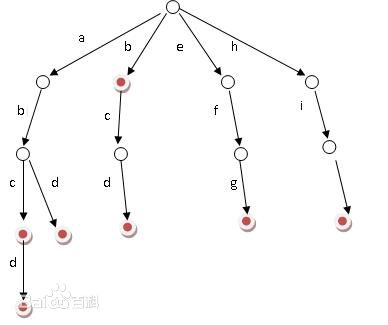# 定义# 实现

## 结构体定义

struct Node{
int val; // 自己根据题目记录的值
Node *next[MAXM]; // MAXM 表示的字符集大小
}*root;


## 插入操作

void insert(char *str){
Node *v = root;
int len = strlen(str+1);
FOR(i,1,len){
int x = str[i] - 'a';
// 如果这个地方没有出现过这个字符，就新建一个
if(!v->next[x]) v->next[x] = New();
v = v->next[x];
// 进入下一层继续构建这个单词
v->val++; // 根据前缀要求这里记录的是答案
}
// v->val++; // 如果是单纯查询单词的话
}


## 询问操作

int calc(char *str){
int len = strlen(str+1);
Node *v = root;
FOR(i,1,len){
int x = str[i]-'a';
if(!v->next[x]) return 0; // 不存在这个前缀
v = v->next[x];
}
return v->val; // 找到这个前缀了
}


# 题目练习

## 代码

#include
#include
#include
#include
#include
#include
#include
#include
#include
#include
#include
#define Re register
#define LL long long
#define U unsigned
#define FOR(i,a,b) for(Re int i = a;i <= b;++i)
#define ROF(i,a,b) for(Re int i = a;i >= b;--i)
#define CLR(i,a) memset(i,a,sizeof(i))
#define BR printf("--------------------\n")
#define DEBUG(x) std::cerr << #x << '=' << x << std::endl

const int MAXN = 100000+5;

struct Node{
struct Edge *first;
int dist;
}node[MAXN];

struct Edge{
Node *s,*t;
Edge *next;int w;
}pool[MAXN<<1],*frog = pool;

Edge *New(Node *s,Node *t,int w){
Edge *ret = ++frog;
*ret = (Edge){s,t,s->first,w};
return ret;
}

inline void add(int u,int v,int w){
node[u].first = New(&node[u],&node[v],w);
node[v].first = New(&node[v],&node[u],w);
}

void dfs(Node *v,Node *fa=NULL){
for(Edge *e = v->first;e;e = e->next){
if(e->t == fa) continue;
e->t->dist = v->dist^e->w;
dfs(e->t,v);
}
}

struct Trie{
Trie *next;
}pool2[MAXN*33],*frog2 = pool2,*root;

Trie *New(){
Trie *ret = ++frog2;
ret->next = ret->next = NULL;
return ret;
}

void insert(int x){
Trie *v = root;
ROF(base,30,0){
int t = (x&(1<>base;
if(!v->next[t]) v->next[t] = New();
v = v->next[t];
}
}

int query(int x){
Trie *v=root;
int ans = 0;
ROF(base,30,0){
int t = (x&(1<>base;
// if(!v->next && !v->next) return 0;
if(!v->next[!t]) v = v->next[t];
else v = v->next[!t],ans += (1<
Last modification：April 7th, 2020 at 10:14 pm